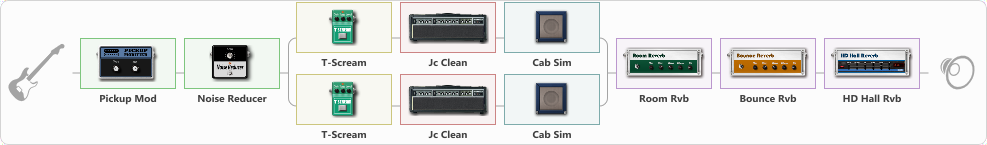# Tasty fuzzy crunch

Discussion in 'ToneLib-GFX presets' started by Konstantinwoe, Apr 12, 2020.

1. Tasty fuzzy crunch

Preset name: Tasty fuzzy crunch for Epi SG

Made on Epiphone G-310, bridge pickup

Effects chain:Effect: "Pickup Mod" (Dynamics / Filter), active - "yes"
{
"Mode" = Humbacker->Single
"Tone" = 28
}

Effect: "Noise Reducer" (Dynamics / Filter), active - "yes"
{
"Sens" = 75
"Mode" = Soft
}

Effect: "Splitter" (Dynamics / Filter), active - "yes"
{
"A-Bypass" = Off
"A-Pan" = 0
"A-Level" = 55
"B-Bypass" = Off
"B-Pan" = 0
"B-Level" = 55

'A' branch:
{

Effect: "T-Scream" (Overdrive / Distortion), active - "yes"
{
"Drive" = 0
"Tone" = 52
"Level" = 80
}

Effect: "Jc Clean" (Amp simulators), active - "yes"
{
"Gain" = 80
"Bass" = 55
"Middle" = 45
"Treble" = 30
"Presence" = 77
"Master" = 100
"Level (dB)" = -4
}

Effect: "Cab Sim" (Cabinets), active - "yes"
{
"Model" = 2x12" Blackface
"Level (dB)" = 0
}
}
'B' branch:
{

Effect: "T-Scream" (Overdrive / Distortion), active - "yes"
{
"Drive" = 0
"Tone" = 52
"Level" = 80
}

Effect: "Jc Clean" (Amp simulators), active - "yes"
{
"Gain" = 80
"Bass" = 55
"Middle" = 45
"Treble" = 30
"Presence" = 77
"Master" = 100
"Level (dB)" = -4
}

Effect: "Cab Sim" (Cabinets), active - "yes"
{
"Model" = 2x10" Vibrolux
"Level (dB)" = 0
}
}
}

Effect: "Room Rvb" (Reverb), active - "yes"
{
"Time" = 2.9
"PreDelay" = 0
"LoDamp" = 46
"HiDamp" = 25
"Mix" = 59
}

Effect: "Bounce Rvb" (Reverb), active - "yes"
{
"Time" = 5.2
"PreDelay" = 0
"LoDamp" = 23
"HiDamp" = 19
"Mix" = 29
}

Effect: "HD Hall Rvb" (Reverb), active - "yes"
{
"Time" = 2.9
"PreLPF" = 44
"PreDelay" = 74
"HiDamp" = 27
"LoGain" = -3.3
"Mix" = 19
}

Note: You will need to download and install the ToneLib-GFX software to use the preset.

File size:
1.1 KB
Views:
2,870